# Stratification, Slip and Cross Diffusion Impacts on Time Depending Convective Stream with Chemical Reaction

Stratification, Slip and Cross Diffusion Impacts on Time Depending Convective Stream with Chemical Reaction

Sivasankaran SivanandamBhuvaneswari Marimuthu Malarselvi Arumugam Ganga Bhose

Department of Mathematics, King Abdulaziz University, Jeddah, Saudi Arabia

Department of Mathematics, Providence College for Women, Coonoor 643 104, India

Corresponding Author Email:
smsivanandam@kau.edu.sa
Page:
581-588
|
DOI:
https://doi.org/10.18280/mmep.060414
18 June 2019
|
Accepted:
22 August 2019
|
Published:
24 December 2019
| Citation

OPEN ACCESS

Abstract:

The purpose of the paper is to explore the slip and cross diffusion impacts on unsteady MHD convective flow past an elongated surface which includes chemical reaction and stratification. The similarity transform is adopted to transfigure the nonlinear PDEs to nonlinear ODEs and then the solution is obtained by Runge-Kutta integration procedure with shooting method. Numerically determined solutions are presented through graphs. The analysis is made for local skin friction, local Nusselt number and local Sherwood number numerically. It is found that thermal slip and thermal stratification diminished the heat transfer rate for both suction/injection.

Keywords:

unsteady flow, MHD, chemical reaction, Soret/Dufour effect, stratification

1. Introduction

Owing to its multifarious practical applications the investigation of the time depending fluid stream with combined mass and energy transport in a chemically reactive viscous fluid stream of an electrically conducting fluid under magnetic field over an elongated sheet has benefited the engineering and scientific domains. The few example of such type of problems are production of plastic and metal sheets, manufacture of wires and paper production etc. To be more particular several metallurgical formations contain the heat reduction of continuous sheets or strips by pulling them over a quiet flow and during the dragging performance these filaments are occasionally elongated and the heat control is essential in the process.

Chamkha et al.  analyzed the interaction of radiation and mixed convection of MHD stream along a permeable surface with Dufour and Soret impacts. It was reported that the existence radiation accelerated the stream energy and diminished the solute concentration. Alam et al.  made a research on the mixed convective stream through a vertical plate with Dufour and Soret influenced including variable suction. They made the conclusion that enhancing values of suction parameter reduced the stream velocity. El-dabe et al.  studied unsteady hydro-magnetic naturally convective stream over a semi-infinite permeable dynamic plate with radiation and chemical reaction. Hakeem et al.  investigated the influence of partial slip on hydro-magnetic stream past an elongated surface with non-uniform thermal source/sink, radiation and wall mass transport. It was concluded that the stream energy was increased by rising porosity values. Sivasankaran et al.  explored the influence of slip and radiation on MHD mixed convective stream with chemical reaction near a stagnation-point flow towards a vertical plate with convective boundary condition. It was reported that the stream velocity increased on decreasing chemical reaction parameter.

Bhuvaneswari et al.  made the Lie group investigation on free convective flow over an inclined surface with internal energy generation and radiation. They noticed that the stream energy was reduced by the rising values of thermal radiation. Kasmani et al.  studied chemically reactive stream with energy convection in a nanofluid past a wedge with thermal source/sink. They reported that the stream energy was reduced by the enhancing chemical reaction. A comparative study for the effects of chemical reaction, radiation and slip on MHD convection stagnation-point flow in a porous medium was done by Niranjan et al. . The impacts of Dufour and Soret on chemically reactive viscoelastic stream with convective boundary condition in an elongated surface which includes mass and heat transport under the existence of radiation were dealt by Eswaramoorthi et al. . Chamkha et al.  considered time depending chemically reactive flow with mass and heat transport from an expanding surface which includes source or sink. It was concluded that the unsteadiness parameter decreased the stream energy. Tsai et al.  analyzed the impact of Dufour and Soret with mass and heat transport on Hiemenz stream onto extended surface in a medium with porosity. They reported that enhancing Prandtl number decreased the stream energy.

Manglesh et al.  examined hydro-magnetic natural convective stream in a medium with porosity under the existence of Hall current, thermal diffusion and radiation. They concluded that the concentration was increased by the enhancing Soret values. Karthikeyan et al.  examined magneto-hydrodynamic mixed convection of a chemically reactive stagnation point stream towards a vertical plate in a medium with porosity which includes radiation and thermal generation under the influence of Soret and Dufour. Beg et al.  considered the problem of natural convection MHD mass and energy transport from an extended surface in a saturated porous medium with Dufour and Soret impacts. It was noticed that the flow was decelerated by the rising magnetic field. MHD convective stream of an extended surface in a porous medium which includes energy and mass transmission and chemical reaction was examined by Mansour et al. , Bhuvaneswari et al. , Kasmani et al. , and Niranjan et al. .

In the all above said studies, the stratification (thermal and solutal) and slip effects are not reported in the unsteady convective flow of chemically reacting fluid in the presence of magnetic field and suction/injection. Hence, the present study intends to investigate the problem of time depending MHD convective stream with suction/injection with stratification, slip, chemical reaction and cross diffusion effects. The formulation of the problem, method of solution, results and discussions are provided in for following sections.

2. Mathematical Formulation

The time depending, 2D laminar stream of a viscous incompressible electrically conducting fluid owing to an extended surface is examined. The sheet is impulsively elongated with variable velocity Uw(x,t) at time t=0. The fluid properties are supposed to be constant. Moreover, the influences of Soret, Dufour, stratification, slip and chemical reaction are considered. It is presumed that the first-order homogeneous chemical reaction takes place in the stream. Using the Boussinesq approximation, the governing equations are drafted as

$\frac{\partial u}{\partial x}+\frac{\partial v}{\partial y}=0$    (1)

$\frac{\partial u}{\partial t}+u \frac{\partial u}{\partial x}+v \frac{\partial u}{\partial y}=v \frac{\partial^{2} u}{\partial y^{2}}+g \beta_{T}\left(T-T_{\infty}\right)+g \beta_{C}\left(C-C_{\infty}\right)-\frac{v}{k_{1}} u-\frac{\sigma B_{0}^{2}}{\rho}\left(u-u_{\infty}\right)$      (2)

$\frac{\partial T}{\partial t}+u \frac{\partial T}{\partial x}+v \frac{\partial T}{\partial y}=\alpha \frac{\partial^{2} T}{\partial y^{2}}+\frac{D_{e} k_{T}}{c_{S} c_{P}} \frac{\partial^{2} C}{\partial y^{2}}$    (3)

$\frac{\partial C}{\partial t}+u \frac{\partial C}{\partial x}+v \frac{\partial C}{\partial y}=D \frac{\partial^{2} C}{\partial y^{2}}-k_{c}\left(C-C_{\infty}\right)+\frac{D_{e} k_{T}}{T_{m}} \frac{\partial^{2} T}{\partial y^{2}}$    (4)

In a stream the velocity factors u and v are forth x and y guidelines. The flow is assumed to be in x-direction. A magnetic field of uniform strength B0 is applied in the transverse direction of the stream. At time t = 0, the sheet is impulsively elongated with the variable velocity Uw (x, t). U, Tand Cdenote velocity, temperature and concentration of the free stream respectively. t represents time, μ, ν, σ, ρ, θ, φ, A and C represent coefficient of viscosity, kinematic viscosity, electrical conductivity, density, dimensionless temperature, dimensionless concentration, unsteadiness parameter, mass concentration respectively. D, cP, cs,, De, f, f', g denote coefficient of mass diffusivity, specific heat, concentration susceptibility, coefficient of effective mass diffusivity, dimensionless stream function, dimensionless velocity, acceleration due to gravity in order. K, k1, kc, kT, mw, p, qw, Tm, Tw, βC, βT stand for permeability parameter, permeability of porous medium, rate of chemical reaction, thermal diffusion ratio, Mass flux, pressure, local wall heat flux, fluid mean temperature, wall temperature, coefficient concentration expansion, coefficient of thermal expansion in order.

The boundary conditions are

$u=u_{w}+L \frac{\partial u}{\partial y} \quad v=v_{w} \quad T=T_{W}+K_{1} \frac{\partial T}{\partial y}$

$C=C_{W}+K_{2} \frac{\partial C}{\partial y} \quad$ at $y=0$     (5)

$\mathrm{u} \rightarrow 0, \quad \mathrm{T} \rightarrow \mathrm{T}_{\infty,} \quad \mathrm{C} \rightarrow \mathrm{C}_{\infty} \quad$ as $\quad \mathrm{y} \rightarrow \infty$

It is presumed that the elongating velocity Uw(x,t), the surface temperature Tw(x,t), and the surface concentration Cw(x,t) are of the form

$U_{w}(x, t)=\frac{a x}{1-c t}, T_{w}=T_{0}+\frac{b_{1} x}{1-c t}, \quad T_{\infty}=T_{0}+\frac{b_{2} x}{1-c t}, C_{w}=C_{0}+\frac{b_{3} x}{1-c t}, C_{\infty}=C_{0}+\frac{b_{4} x}{1-c t}$   (6)

where, a, b1, b2, b3, b4 are constants with ct < 1. VW is the suction/injection parameter, VW > 0 (injection) and VW < 0 (suction). The following similarity transformation is applied in this work.

$\psi=\sqrt{\frac{a v}{(1-c t)}} x f(\eta), \eta=\sqrt{\frac{a}{v(1-c t)}} y$

$T=T_{\infty}+\left(T_{W}-T_{0}\right) \theta(\eta), C=C_{\infty}+\left(C_{W}-C_{0}\right) \phi(\eta)$    (7)

ψ is the stream function $\mathrm{u}=\frac{\partial \psi}{\partial v} \& v=-\frac{\partial \psi}{\partial x}$, so that continuity Eq. (1) is satisfied. Using the above transformation Eqns. (2)-(5) reduced to

$f^{\prime \prime\prime}(\eta)+R_{i T}[\theta+N \varphi]-\left[f^{\prime}(\eta)\right]^{2}+f(\eta) f^{\prime \prime}(\eta)-A\left[f^{\prime}(\eta)+\frac{1}{2} \eta f^{\prime \prime}(\eta)\right]-M f^{\prime}(\eta)=0$    (8)

$\frac{1}{\operatorname{Pr}} \theta^{\prime \prime}(\eta)+D f \varphi^{\prime \prime}(\eta)-A\left[\frac{1}{2} \eta \theta^{\prime}(\eta)+\theta(\eta)\right]+f(\eta) \theta^{\prime}(\eta)-f^{\prime}(\eta) \theta(\eta)-S t_{T} f^{\prime}-A S t_{T}=0$    (9)

$\frac{1}{S c} \varphi^{\prime \prime}(\eta)-C r \phi(\eta)-f^{\prime}(\eta) \varphi(\eta)+f(\eta) \varphi^{\prime}(\eta)-A\left[\frac{1}{2} \eta \varphi^{\prime}(\eta)+\varphi(\eta)\right]+S r \theta^{\prime \prime}(\eta)-S t_{C} f^{\prime}-A S t_{C}=0$    (10)

The dimensionless boundary conditions (5) becomes

$f^{\prime}(\eta)=1+d f^{\prime \prime}(\eta) \quad f(\eta)=f_{w}$

$\theta(\eta)=1-S t_{T}+d_{T} \theta^{\prime}(\eta)$

$\phi(\eta)=1-S t_{C}+d_{T} \phi^{\prime}(\eta) \quad$ at $\eta=0$

$f^{\prime}(\eta) \rightarrow 0 \quad \theta(\eta) \rightarrow 0 \quad \phi(\eta) \rightarrow 0 \quad$ as $\eta \rightarrow \infty$    (11)

where, $G r_{T}=g \beta_{T}\left(T_{W}-T_{\infty}\right) x^{3} / v^{2} \quad, \quad G r_{C}=g \beta_{C}\left(C_{W}-\right.\left.C_{\infty}\right) x^{3} / v^{2}, R i_{T}=\frac{G r_{T}}{R e^{2}}, \quad R i_{C}=\frac{G r_{C}}{R e^{2}}, \quad N=\frac{R i_{C}}{R i_{T}}$ represent thermal Grashof number, solutal Grashof number, thermal Richardson number, solutal Richardson number respectively. $H a^{2}=\frac{\sigma B_{0}^{2} x^{2}}{\rho y}$ Hartmann number, $M=K+\frac{H a^{2}}{R e}$ porous magnetic parameter, $S c=\frac{v}{D}$ Schmidt number, $A=\frac{c}{a}$ unsteadiness parameter, $\operatorname{Pr}=\frac{\mu c_{p}}{k}$ Prandtl number, $R e=\frac{U_{w} x}{v}$ local Reynolds number, $D f=\frac{D e k_{T} b_{3}}{c_{s} c_{p} v b_{1}}$ Dufour number, $S r=\frac{\operatorname{Dek}_{T}\left(T_{W}-T_{\infty}\right)}{c_{S} c_{p} v\left(C_{W}-C_{\infty}\right)}$ Soret number, $C r=\frac{k_{c}(1-c t)}{a}$ chemical reaction parameter, $d=\sqrt{\frac{u_{w}}{v x}}$ velocity slip parameter, $d_{T}=K_{1} \sqrt{\frac{u_{w}}{v x}}$ thermal slip parameter, $d_{C}=K_{2} \sqrt{\frac{u_{w}}{v x}}$ solutal slip parameter, $S t_{T}=\frac{b_{2}}{b_{1}}$ thermal stratification parameter, $S t_{C}=\frac{b_{4}}{b_{3}}$ solutal stratification parameter.

The non-dimensional form of the skin drag magnitude at the wall is defined as $C f_{x}=\frac{\mu\left(\frac{\partial u}{\partial y}\right)_{y=0}}{\rho\left(u_{w}\right)^{2}}$ we get $\frac{1}{2} C f_{x} \sqrt{\operatorname{Re} x}=-f^{\prime \prime}(0).$ The Nusselt number is drafted as $N u x=\frac{x q_{w}}{k\left(T_{w}-T_{\infty}\right)}$ where $q_{w}=-K\left(\frac{\partial T}{\partial y}\right)_{y=0},$ we get $N u x=-\theta^{\prime}(0) \sqrt{\operatorname{Re} x} .$ The local Sherwood number is given by $Shx=\frac{x m_{w}}{D\left(C_{W}-C_{\infty}\right)}$ where $m_{w}=D\left(\frac{\partial c}{\partial y}\right)$ at $y=0$ using the abovel result it is obtained as $S h x=-\phi^{\prime}(0) \sqrt{R e x}$

3. Results and Discussion

The system of nonlinear ODEs (8)-(10) with boundary conditions (11) are solved by utilizing shooting procedure with Runge-Kutta integration method. The comparison is very important in the numerical calculations. The present results for some particular cases are compared with the numerical results available in literature [10, 19] and it is shown in Table 1 for $R i=S_{T}=S_{C}=d_{T}=d=0$. The numerical results obtained in the present work agreed well with the available results.

Table 1. Comparison of numerical results of $-f^{\prime \prime}(0)$ with $f w=0, R i=S t_{T}=S t_{C}=M=d_{T}=d=0$

 A Sharidan et al.  Chamkha et al.  Present work 0.8 1.261042 1.261512 1.261305 1.2 1.377722 1.378052 1.377914

It is inferred from the Figure 1 that velocity slip values reduce the stream speed. Figure 2 shows the increasing values of thermal slip parameter produce decrement in thermal boundary layer. Figure 3 exhibits that increasing values of solutal slip parameter have the tendency to pull down the concentration. Figure 4 shows the enhancing values of thermal stratification parameter diminish the stream temperature. Figure 5 discloses that the solutal stratification values decelerate the concentration boundary layer. Figure 6 shows the raising values of chemical reaction parameter decrease stream concentration. Figure 7 exhibits increasing Soret values raise the concentration boundary layer. It is inferred through the figures 8(a) & 8(b) that the Dufour values induce increment in both the velocity and fluid temperature.

## 1.jpgFigure 1. Effects of velocity slip parameter d on velocity

## 2.jpg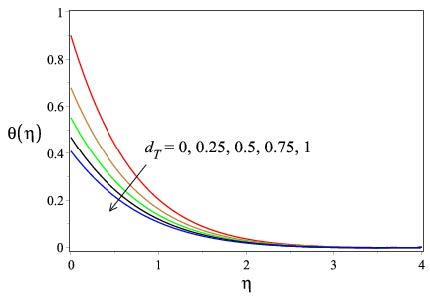Figure 2. Effects of thermal slip parameter dT on temperature

## 3.jpg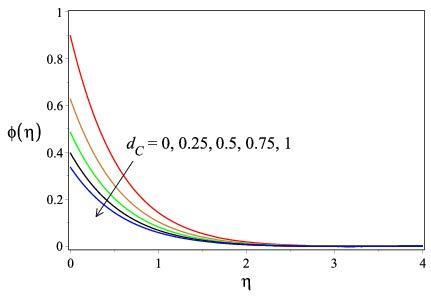Figure 3. Effects of solutal slip parameter on concentration

## 4.jpgFigure 4. Effects of thermal stratification StT on temperature

## 5.jpg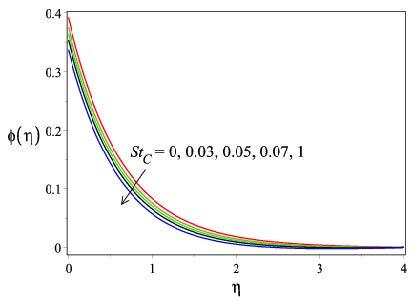Figure 5. Effects of solutal stratification StC on concentration

## 6.jpgFigure 6. Effects of chemical reaction on concentration

## 7.jpgFigure 7. Effects of Soret parameter Sr on concentration

The parametric inspection is implemented to analyse the characteristics of skin drag, heat transfer rate and solute transmission rate through graphs. The influence of thermal slip with suction/injection on skin friction, Nusselt number, Sherwood number against thermal stratification is noticed through Figure 9(a)-9(c). It is understood that enhancing values of thermal slip values against thermal stratification improve the skin drag magnitude in both cases of suction and injection and the Nusselt number is diminished. The solute transfer rate is decelerated by the thermal slip and thermal stratification values in the case of suction but is accelerated by the lower thermal stratification with high thermal slip values in the case of injection. Figure 10(a) indicates that higher solutal slip values and lower Soret values increase the magnitude of skin friction. Figure 10(b) & Figure 10(c) inform that increasing values of both the solutal slip and Soret parameter boost the heat transfer rate for suction/injection but they decrease the local Sherwood number. Figure 11(a) exhibits the behaviour of change in the magnitude of skin drag verses the Dufour parameter for the values of thermal slip. It is examined that the values of skin drag are raised by the higher thermal slip values with lower Dufour numerals.

## 8a.jpg(a)

## 8b.jpg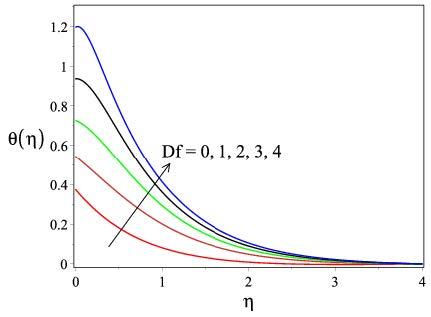(b)

Figure 8. Effects of Dufour parameter Df on (a) velocity, (b) temperature

## 9a.jpg(a)

## 9b.jpg(b)

## 9c.jpg(c)

Figure 9. Influence of thermal slip with fw on (a) skin friction, (b) Nusselt number, (c) Sherwood number against thermal stratification with d = 1.0; Stc = 0.1; dc = 1.0; A = 0.1; M = 0.1; Cr = 1.0; Df = 0.2; Sr = 0.2.

## 10a.jpg(a)

## 10b.jpg(b)

## 10c.jpg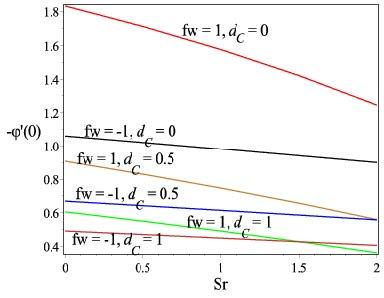(c)

Figure 10. Influence of solutal slip with fw on (a) skin friction, (b) Nusselt number, (c) Sherwood number against Soret number with d = 1.0; StT = 0.1.; dt = 1.0; Stc = 0.1; A = 0.1; M = 0.1; Cr = 1.0; Df = 0.2

Figure 11(b) shows that the Nusselt number is decelerated by the enhancing values of Dufour parameter and thermal slip parameter with suction/injection. Fig. 11(c) shows the values of Sherwood increases by rising numerals of thermal slip parameter and Dufour parameter in the case of injection but it is decelerated by higher thermal slip values with lower Dufour parameter for suction. It is observed from the Fig. 12(a) that the skin drags magnitude is improved by the increasing values of solutal stratification with decreasing Soret values for both suction and injection. Nusselt number variation is observed significantly for different solutal stratification and Soret parameter through Figure 12 (b). It is understood that Nusselt number increases with increasing solutal stratification and Soret parameter for injection but it is diminished for lower Soret and higher solutal stratification values for suction. Figure 12(c) illustrates that the solute transfer rate is decreased by both solutal stratification and Soret effect for suction/injection.

## 11a.jpg(a)

## 11b.jpg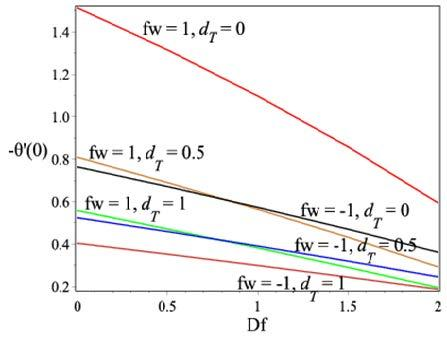(b)

## 11c.jpg(c)

Figure 11. Influence of thermal slip with fw on (a) skin friction, (b) Nusselt number, (c) Sherwood number against Dufour number with d = 1.0; StT= 0.1.; StC = 0.1; dc = 1.0; A = 0.1; M = 0.1; Cr = 1.0; Sr = 0.2

## 12a.jpg(a)

## 12b.jpg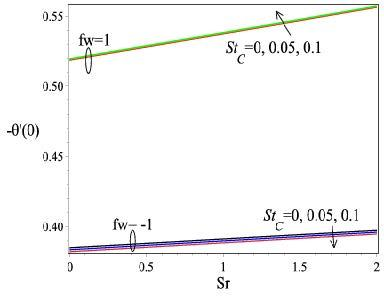(b)

## 12c.jpg(c)

Figure 12. Influence of solutal stratification with fw on (a) skin friction, (b) Nusselt number, (c) Sherwood number against Soret number with d = 1.0; StT = 0.1.; dt = 1.0; dc = 1.0; A = 0.1; M = 0.1; Cr = 1.0; Df = 0.2

## 13a.jpg(a)

## 13b.jpg(b)

## 13c.jpg(c)

Figure 13. Influence of thermal stratification with fw on (a) skin friction, (b) Nusselt number, (c) Sherwood number against Dufour number with d = 1.0; dt = 1.0; StC = 0.1; dc = 1.0; A = 0.1; M = 0.1; Cr = 1.0; Sr = 0.2

## 14a.jpg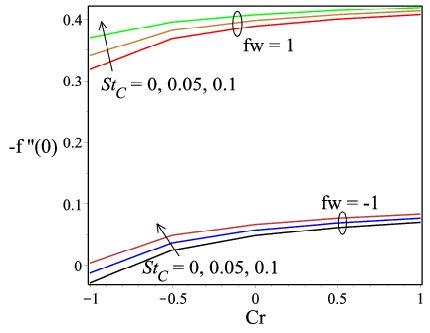(a)

## 14b.jpg(b)

## 14c.jpg(c)

Figure 14. Influence of solutal stratification with fw on (a) skin friction, (b) Nusselt number, (c) Sherwood number against chemical reaction parameter with d = 1.0; StT = 0.1; dT = 1.0; dc = 1.0; A = 0.1; M = 0; Df = 0.2; Sr = 0.2

## 15a.jpg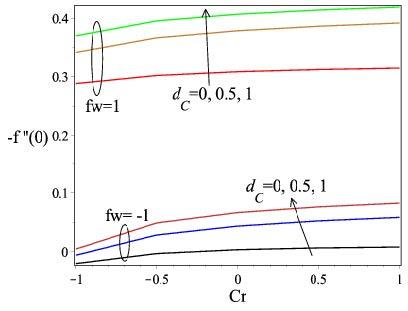(a)

## 15b.jpg(b)

## 15c.jpg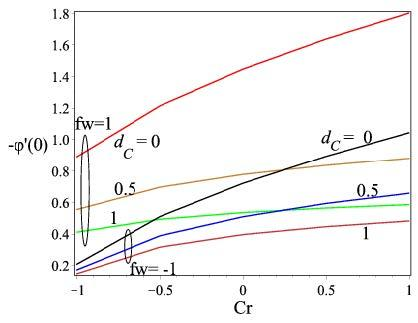(c)

Figure 15. Influence of solutal slip with fw on (a) skin friction, (b) Nusselt number, (c) Sherwood number against chemical reaction parameter with d = 1.0; StT = 0.1; dT = 1.0; Stc = 0.1; A = 0.1; M = 0.1; Df = 0.2; Sr = 0.2

Figure 13(a) shows the skin friction magnitude is accelerated by higher thermal stratification parameter with lower Dufour values for suction/injection. Figure 13(b) gives the information that the Nusselt number is decelerated by both the enhancing values thermal stratification values and Dufour parameter in the existence of suction/injection. Figure 13(c) demonstrates that the Sherwood number is decelerated for lower Dufour values and higher thermal stratification parameter in the existence of suction/injection. It is interesting to notice through Figure 14 (a) that the skin friction becomes higher for higher solutal stratification with increasing chemical reaction parameter for suction/injection. It is evident through Figure 14(b) the Nusselt number becomes lower for both the higher values of solutal stratification and chemical reaction parameter in the presence of suction/injection. Figure 14(c) portrays that the Sherwood number is accelerated by the higher chemical reaction parameter with simultaneous lower solutal stratification under the existence of suction/injection.

Figure 15(a) portrays the domination of solutal slip on skin drag against the chemical reaction parameter with suction/injection. It is clear that the coefficient of skin friction is boosted by the incremented values of solutal slip and chemical reaction parameter with suction/injection. Figure 15(b) clearly gives the information that heat transfer rate is decreased by the higher values of chemical reaction parameter and lower solutal slip in the existence of suction/injection. It is understood through Figure 15(c) that the Sherwood number becomes lower for higher solutal slip with lower chemical reaction parametric values under suction/injection.

4. Conclusion

Thermal slip and thermal stratification enhance the skin drag and they diminish the local Nusselt number for both suction/injection. Enhancing thermal slip with diminishing thermal stratification increase the solute transfer rate in the case of injection but the rate of solute transfer is decreased by the higher thermal slip and thermal stratification parameters in the consideration of suction.

Rising solutal slip with decreasing Soret values improve skin friction. Nusselt number is enhanced by both solutal slip and Soret values with suction/injection. Sherwood number is decelerated by the enhancing values of both solutal slip parameter and Soret values.

Higher solute stratification with lower Soret values increases the magnitude of skin drag for both suction/injection. Both the solute stratification and Soret values increase the Nusselt number rate in the case of injection, but higher solutal stratification with lower Soret values diminish the Nusselt number for suction. Raising values of both solutal stratification and Soret values decline the local Sherwood number with suction/injection.

Increasing thermal stratification with diminishing Dufour induce increment in skin friction in the presence of suction/injection. Thermal stratification and Dufour together imply reduction in the local Nusselt number for both suction/injection. Higher Dufour with lower thermal stratification induce increment in the solute transfer rate for suction/injection.

Slip values opposes the stream velocity. The thermal slip pulls down thermal boundary layer and solutal slip decelerates concentration. Thermal stratification reduces the fluid temperature. Solute stratification diminishes the concentration boundary layer. Chemical reaction parameter reduces the concentration boundary layer. Soret values increase the fluid concentration. Dufour parameter induces increment in the velocity and temperature.

References

 Chamkha, A.J., Ben-Nakhi, A.L. (2008). MHD mixed convection-radiation interaction along a permeable surface immersed in a porous medium in the presence of Soret and Dufour’s effects. Heat and Mass Transfer, 44(2008): 845-856. https://doi.org/10.1007/s00231-007-0296-x

 Alam, M.S., Rahman, M.M. (2006). Dufour and Soret effects on mixed convection flow past a vertical porous flat plate with variable suction. Nonlinear Analysis: Modelling and Control, 11(1): 3-12.

 El-dabe, N.T.M., Hassan, M.A., Godh, W.A. (2013). Unsteady magnetohydrodynamic free convection flow past a semi-infinite permeable moving plate through porous medium with chemical reaction and radiation absorption. Journal of Heat Transfer, 135: 024501-(1-5). https://doi.org/10.1115/1.4007474

 Hakeem, A.K.A., Kalaivanan, R., Ganesh, N.V., Ganga, B. (2014). Effect of partial slip on hydromagnetic flow over a porous stretching sheet with non-uniform heat source/sink. Thermal Radiation and Wall Mass Transfer, Ain Shams Engineering Journal, 5: 913-922. https://doi.org/10.1016/j.asej.2014.02.006

 Sivasankaran, S., Niranjan, H., Bhuvaneswari, M. (2017). Chemical reaction, radiation and slip effects on MHD mixed convection stagnation point-flow in a porous medium with convective boundary condition. International Journal of Numerical Methods for Heat & Fluid Flow, 27(2): 454-470. https://doi.org/10.1108/HFF-02-2016-0044

 Bhuvaneswari, M., Sivasankaran, S., Kim, Y.J. (2012). Lie group analysis of radiation natural convection flow over an inclined surface in a porous medium with internal heat generation. Journal of Porous Media, 15(12): 1155-1164. https://doi.org/10.1615/JPorMedia.v15.i12.80

 Kasmani, R.M., Sivasankaran, S., Bhuvaneswari, M., Siri, Z. (2016). Effect of chemical reaction on convective heat transfer of boundary layer flow in nanofluid over a wedge with heat generation/absorption and suction. Journal of Applied Fluid Mechanics, 9 (1): 379-388. https://doi.org/10.18869/acadpub.jafm.68.224.24151

 Niranjan, H., Sivasankaran, S., Bhuvaneswri, M. (2016). Analytical and numerical study on magneto convection stagnation-point flow in a porous medium with chemical reaction, radiation and slip effects. Mathematical Problems in Engineering, Article ID 4017076.

 Eswaramoorthi, E., Bhuvaneswari, M., Sivasankaran, S., Rajan, S. (2016). Soret and Dufour effects of viscoelastic boundary layer flow, heat and mass transfer in a stretching surface with convective boundary condition in the presence of radiation and chemical reaction. Scientia Iranica B, 23(6): 2575-86. https://doi.org/10.24200/SCI.2016.3967

 Chamkha, A.J., Aly, A.M., Mansour, M.A. (2010). Similarity solution for Unsteady heat and mass transfer from a stretching surface embedded in a porous medium with suction/injection and chemical reaction effects. Chemical Engineering Communications, 197: 846-858. https://doi.org/10.1080/00986440903359087

 Tsai, R., Huang, J.S. (2009). Heat and mass transfer for Soret and Dufour’s effects on Hiemenz flow through porous medium onto a stretching surface. International Journal of Heat and Mass Transfer, 52: 2399-2406. https://doi.org/10.1016/j.ijheatmasstransfer.2008.10.017

 Manglesh, A., Gorla, M.G. (2013). MHD Free convective flow through porous medium in the presence of hall current, radiation and thermal diffusion. Indian Journal of Pure and Applied Mathematics, 44(6): 743-756. https://doi.org/10.1007/s13226-013-0040-9

 Karthikeyan, S., Bhuvaneswari, M., Sivasankaran, S., Rajan, S. (2016). Soret and Dufour effects on MHD mixed convection heat and mass transfer of a stagnation point flow towards a vertical plate in a porous medium with chemical reaction, radiation and heat generation. Journal of Applied Fluid Mechanics, 9(3): 1447-1455. https://doi.org/10.18869/acadpub.jafm.68.228.24135

 Beg, O.A., Prasad, V.R., Vasu, B., Reddy, N.B., Li, Q., Bhargava, R. (2011). Free convection heat and mass transfer from an isothermal sphere to a micropolar regime with Soret/Dufour effects. International Journal of Heat and Mass Transfer, 54: 9-18. https://doi.org/10.1016/j.ijheatmasstransfer.2010.10.005

 Mansour, M.A., EL-Anssary, N.F., Aly, A.M. (2008). Effect of chemical reaction and thermal stratification on MHD free convective heat and mass transfer over a vertical stretching surface embedded in a porous media considering Soret and Dufour numbers. Chemical Engineering Journal, 145: 340-345. https://doi.org/10.1016/j.cej.2008.08.016

 Bhuvaneswari, M., Sivasankaran, S., Ferdows, M.(2009). Lie group analysis of natural convection heat and mass transfer in an inclined surface with chemical reaction. Non-Linear Analysis: Hybrid Systems, 3(4): 536-542. https://doi.org/10.1016/j.nahs.2009.04.004

 Kasmani, R.M., Sivasankaran, S., Bhuvaneswari, M., Hussein, A.K. (2017). Analytical and numerical study on convection of nanofluid past a moving wedge with Soret and Dufour effects. International Journal of Numerical Methods Heat & Fluid Flow, 27: 2333-2354. https://doi.org/10.1108/HFF-07-2016-0277

 Niranjan, H., Sivasankaran, S., Bhuvaneswari, M. (2017). Chemical reaction, soret and dufour effects on MHD mixed convection stagnation point flow with radiation and slip condition. Scientia Iranica – Transactions. B: Mechanical Engineering, 24: 698-706. https://doi.org/10.24200/SCI.2017.4054

 Sharidan, S., Mahmood, T., Pop, I. (2006). Similarity solutions for the unsteady boundary layer flow and heat transfer due to a stretching sheet. Int. J. Appl. Mech. Eng., 11: 647–654.